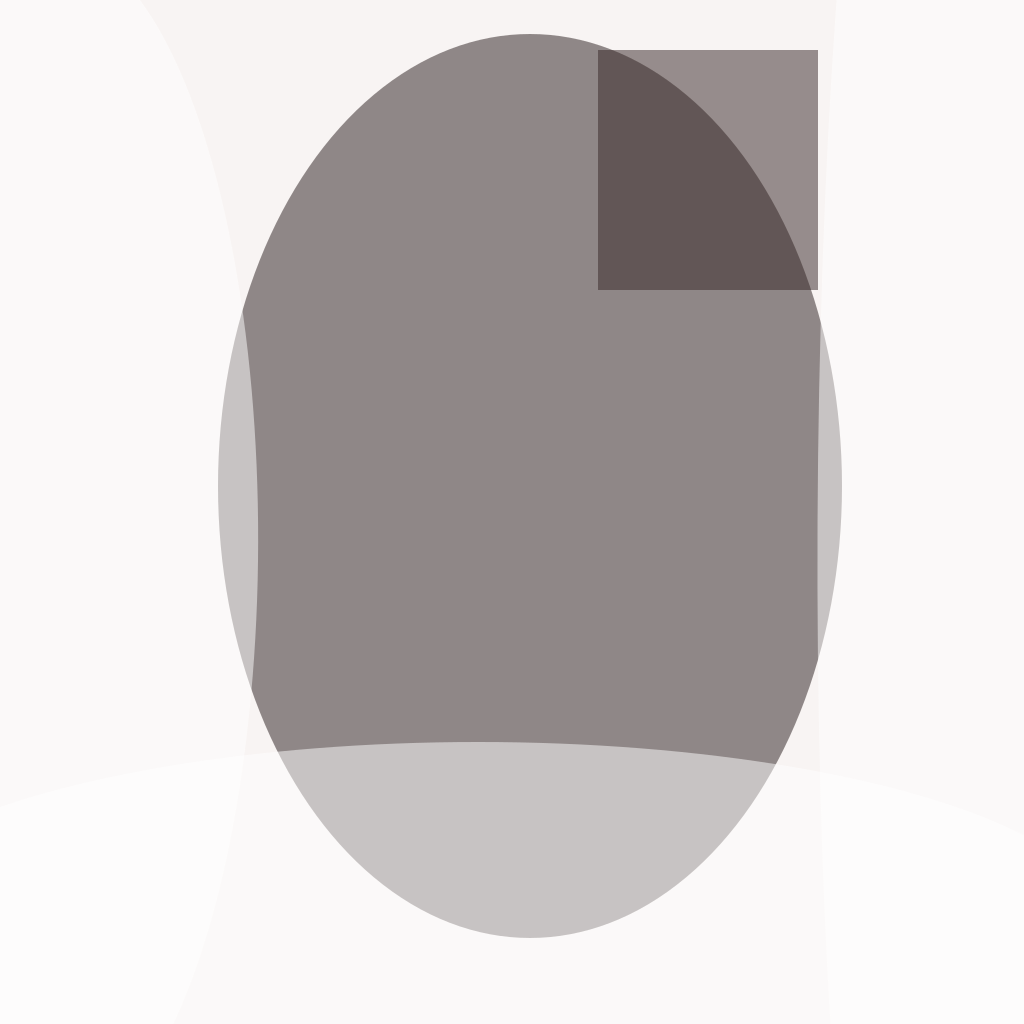# A First Book in Algebra

AU\$8.95AU\$41.95

A comprehensive introductory textbook that covers the fundamental concepts of algebra.A First Book in Algebra – SoftcoverA First Book in Algebra – HardcoverA First Book in Algebra – PDF
SKU: N/A Category:

A First Book in Algebra is a comprehensive introductory textbook that covers the fundamental concepts of algebra. The book includes numerous examples and exercises, as well as clear explanations of mathematical concepts.

Whether you’re a beginner or a more advanced student, A First Book in Algebra is an essential resource for mastering algebra and building a strong foundation in mathematics. Please note that due to the complexity of reformatting mathematical equations, this books has been produced by cleaning a scan I personally made, and then carefully copy/pasting information where the original printer had missing or misformed print.

Book Size 5.5×8.5 B&W Matte None 390 Creme 74gsm Durell, Fletcher Softcover, Hardcover, PDF Maths